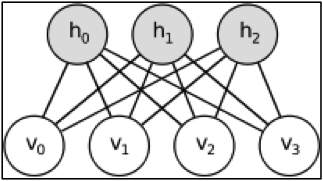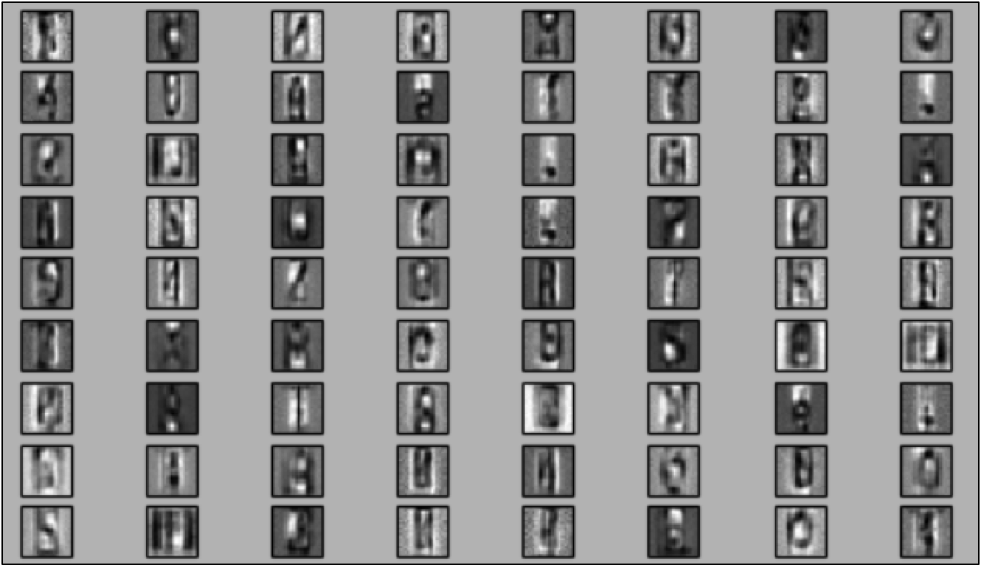﻿ TSM - Restricted Boltzmann Machines
EDITING BOARDRO
EN
×
▼ BROWSE ISSUES ▼

# Restricted Boltzmann MachinesRoland Szabo
Junior Python Developer
@3Pillar Global
PROGRAMMING

In the last article I presented a short history of deep learning and I listed some of the main techniques that are used. Now I"m going to present the components of a deep learning system.

Deep learning had its first major success in 2006, when Geoffrey Hinton and Ruslan Salakhutdinov published the paper "Reducing the Dimensionality of Data with Neural Networks", which was the first efficient and fast application of Restricted Boltzmann Machines (or RBMs).

As the name suggests, RBMs are a type of Boltzmann machines, with some constraints. These have been proposed by Geoffrey Hinton and Terry Sejnowski in 1985 and they were the first neural networks that could learn internal representations (models) of the input data and then use this representation to solve different problems (such as completing images with missing parts). They weren"t used for a long time because, without any constraints, the learning algorithm for the internal representation was very inefficient.

According to the definition, Boltzmann machines are generative stochastic recurrent neural networks. The stochastic part means that they have a probabilistic element to them and that the neurons that make up the network are not fired deterministically, but with a certain probability, determined by their inputs. The fact that they are generative means that they learn the joint probability of input data, which can then be used to generate new data, similar to the original one.

But there is an alternative way to interpret Boltzmann machines, as being energy based graphical models. This means that for each possible input we associate a number, called the energy of the model, and for the combinations that we have in our data we want this energy to be as low as possible, while for other, unlikely data, it should be high.The graphical model for an RBM with 4 input units and 3 hidden units

The constraint imposed by RBMs is that neurons must form a bipartite graph, which in practice is done by organizing them into two separate layers, a visible one and a hidden one, and the neurons from each layer have connections to the neurons in the other layer and not to any neuron in the same layer. In the above figure, you can see that there are no connections between any of the h"s, nor any of the v"s, only between every v with every h.

The hidden layer of the RBM can be thought to be made of latent factors that determine the input layer. If, for example, we analyze the grades users give to some movies, the input data will be the grades given by a certain user to the movies, and the hidden layer will correspond to the categories of movies. These categories are not predefined, but the RBM determines them while building its internal model, grouping the movies in such a way that the total energy is minimized. If the input data are pixels, then the hidden layer can be seen as features of objects that could generate those pixels (such as edges, corners, straight lines and other differentiating traits).

If we regard the RBMs as energy based models, we can use the mathematical apparatus used by statistical physics to estimate the probability distributions and then to make predictions. Actually, the Boltzmann distribution from modeling the atoms in a gas gave the name to these neural networks.

The energy of such a model, given the vector v (the input layer), the vector h (the hidden layer), the matrix W (the weights associated with the connections between each neuron from the input layer and the hidden one) and the vectors a and b (which represent the activations thresholds for each neuron, from the input layer and from the hidden layer) can be computed using the following formula:

The formula is nothing to be scared of, it"s just a couple of matrix additions and multiplications.

Once we have the energy for a state, its probability is given by:

where Z is a normalization factor.

And this is where the constraints from the RBM help us. Because the neurons from the visible layer are not connected to each other, it means that for a given value of the hidden layer neuron, the visible ones are conditionally independent of each other. Using this we can easily get the probability for some input data, given the hidden layer:

where

is the activation probability for a single neuron:

is the logistic function.

In a similar way we can define the probability for the hidden layer, having the visible layer fixed.

How does it help us if we know these probabilities?

Let"s presume that we know the correct values for the weights and the thresholds of an RBM and that we want to determine what items are in an image. We set the pixels of the image as the input of the RBM and we calculate the activation probabilities of the hidden layer. We can interpret these probabilities as filters learned by the RBM about the possible objects in the images.

We take the values of those probabilities and we enter them into another RBM as input data. This RBM will also give out some other probabilities for its hidden layer, and these probabilities are also filters for its own inputs. These filters will be of a higher level and more complex. We repeat this a couple of times, we stack the resulting RBMs and, on top of the last one, we add a classification layer (such as logistic regression) and we get ourselves a Deep Belief Network.

Greedy layerwise training of a DBN

The idea that started the deep learning revolution was this: you can learn layer by layer filters that get more and more complex and at the end you don"t work directly with pixels, but with high level features, that are much better indicators of what objects are there in an image.

The learning of the parameters of a RBM is done using an algorithm called "contrastive divergence". This starts with an example from the input data, calculates the values for the hidden layer and then these values are used to simulate what input data they would produce. The weights are then adjusted with the difference between the original input data and the "dreamed" input data (with some inner products around there). This process is repeated for each example of the input data, several times, until either the error is small enough or a predetermined number of iterations have passed.

There are many implementations of RBMs in machine learning libraries. One such library is scikit-learn, a Python library used by companies such as Evernote and Spotify for their note classifications and music recommendation engines. The following code shows how easy it is to train an RBM on images that each contain one digit or one letter and then to visualize the learned filters.

``````from sklearn.neural_network import BernoulliRBM as RBM
import numpy as np
import matplotlib.pyplot as plt
import cPickle

X = (X - np.min(X, 0)) / (np.max(X, 0) + 0.0001)  # 0-1 scaling
rbm = RBM(n_components=900, learning_rate=0.05, batch_size=100, n_iter=50)
print("Init rbm")

rbm.fit(X)

plt.figure(figsize=(10.2, 10))
for i, comp in enumerate(rbm.components_):
plt.subplot(30, 30, i + 1)
plt.imshow(comp.reshape((20, 20)), cmap=plt.cm.gray_r,
interpolation="nearest")
plt.xticks(())
plt.yticks(())
plt.suptitle("900 components extracted by RBM", fontsize=16)

plt.show()
``````Some of the filters learned by the RBM: you can notice filters for the letters B, R, S, for the digits 0, 8, 7 and some others

Some of the filters learned by the RBM: you can notice filters for the letters B, R, S, for the digits 0, 8, 7 and some others

Some of the filters learned by the RBM: you can notice filters for the letters B, R, S, for the digits 0, 8, 7 and some others

RBMs are an essential component from which deep learning started and are one of the few models that allow us to learn efficiently an internal representation of the problem we want to solve. In the next article, we will see another approach in learning representations, using autoencoders.

## VIDEO: ISSUE 97 LAUNCH EVENT## Roland Szabo wrote also